Welcome to Gaia! :: View User's Journal | Gaia Journals

View User's Journal

~My Art Collection~
avi art people created for me ^.^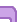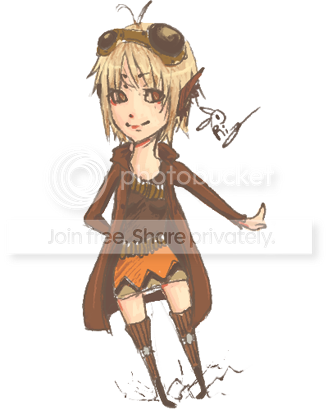Art For My Newest Avi!Thank you to all the artists that contributed to my collection!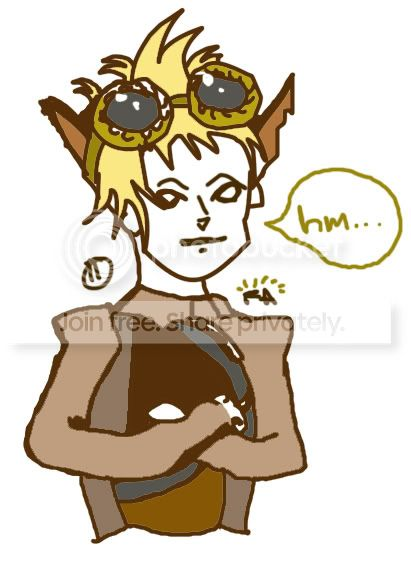^^^This is by long-haired cat^^^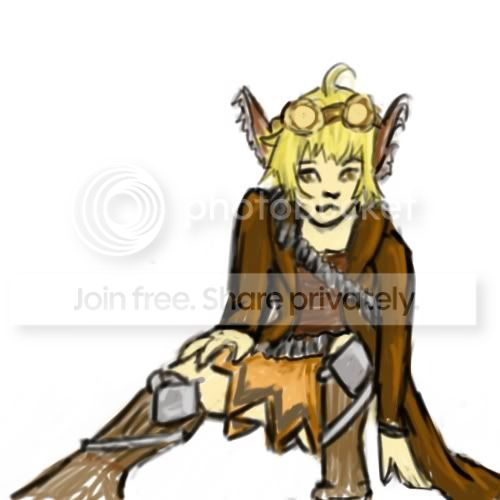^^^This is by La Merwaffle^^^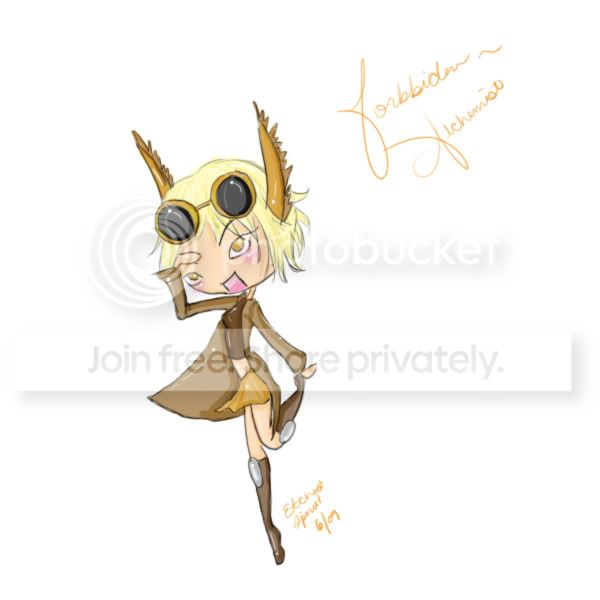^^^This is by EtchedSpirit^^^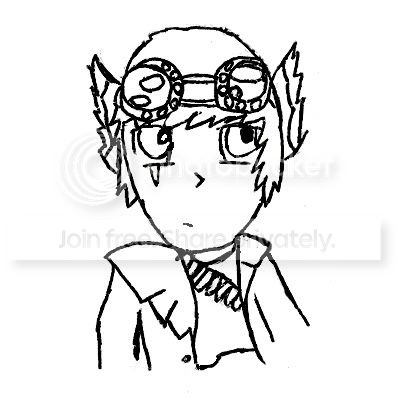^^^This is by dulceice^^^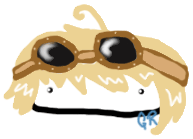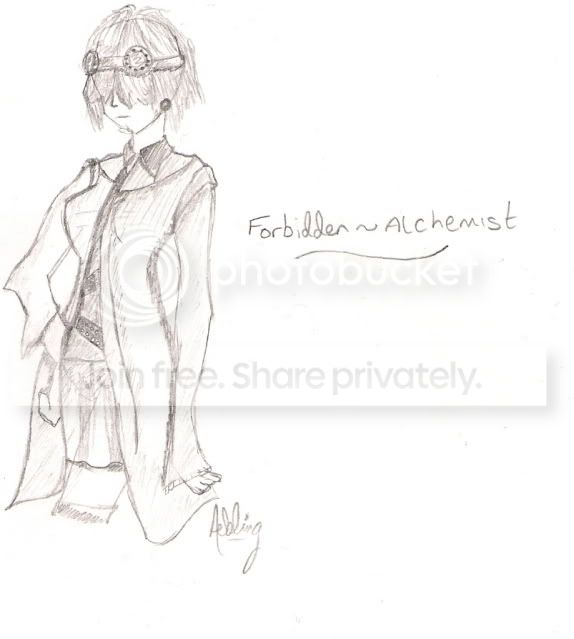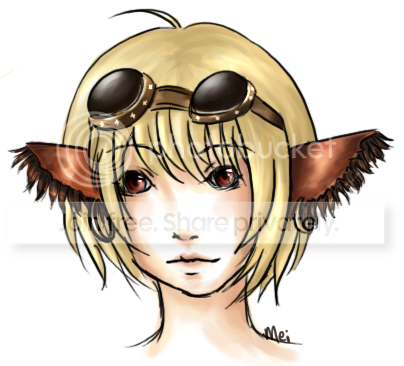^^^This is by MeiXian^^^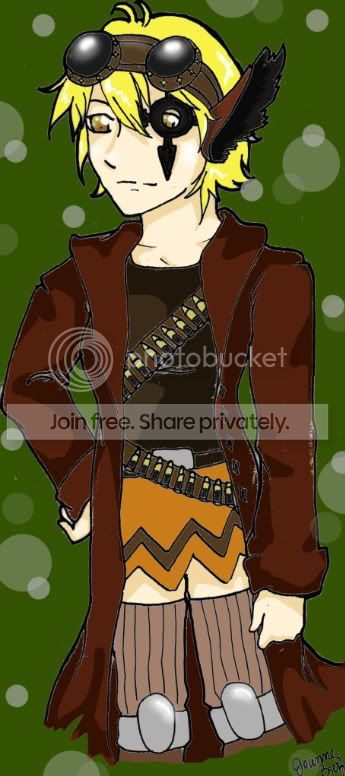^^^This is by joannebb14^^^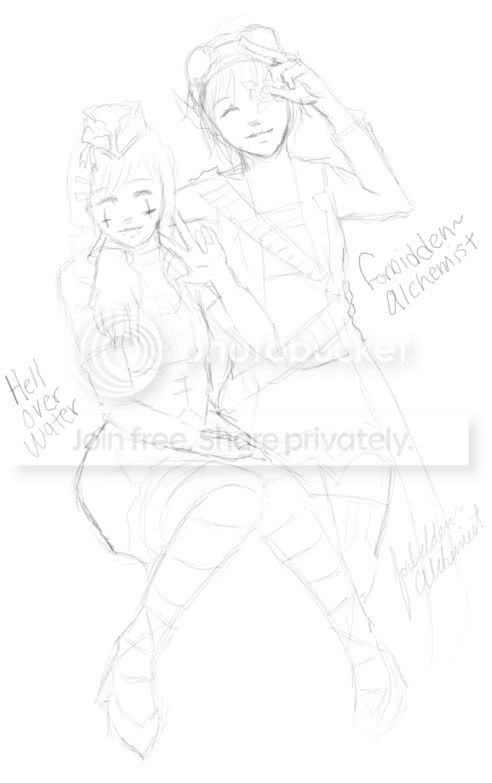^^^This art was made by me^^^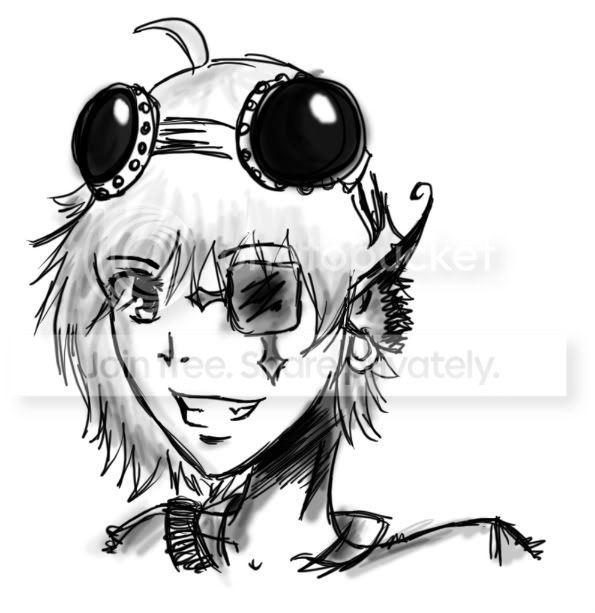^^^This is by Keessa^^^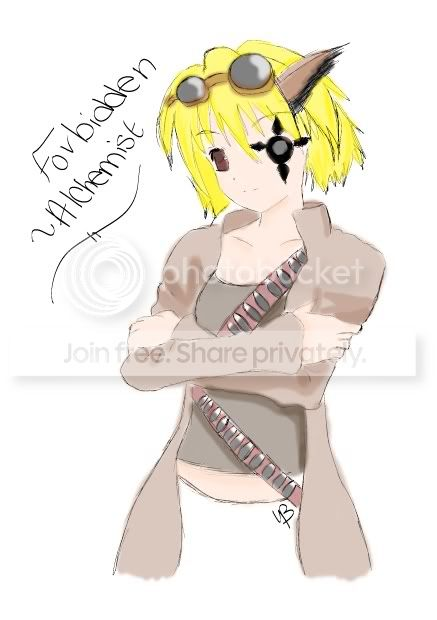^^^This is by YoZaKuRa BlOsSoM^^^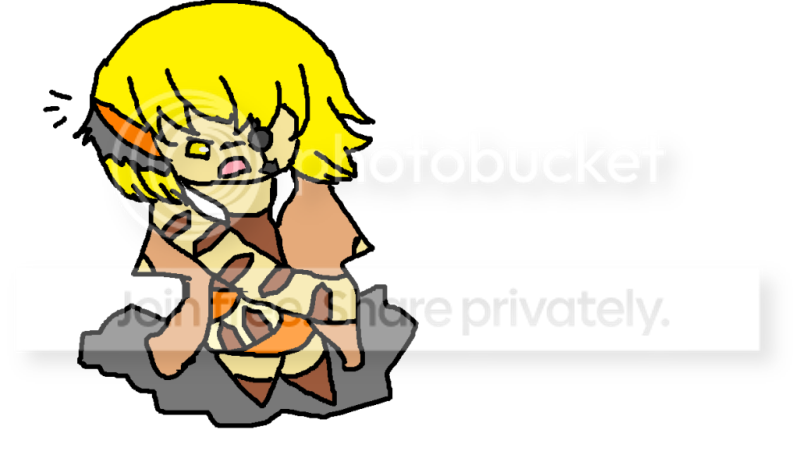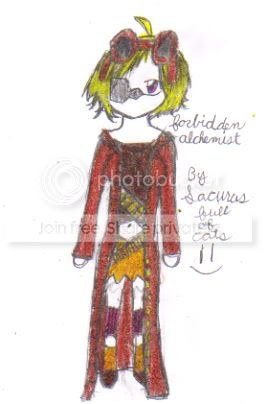^^^This is by sakuras-full-of-cats^^^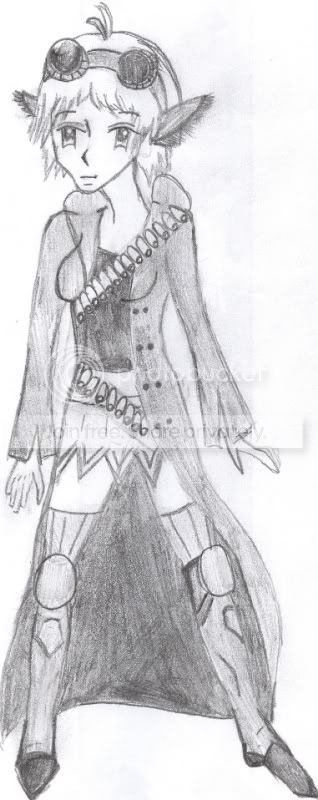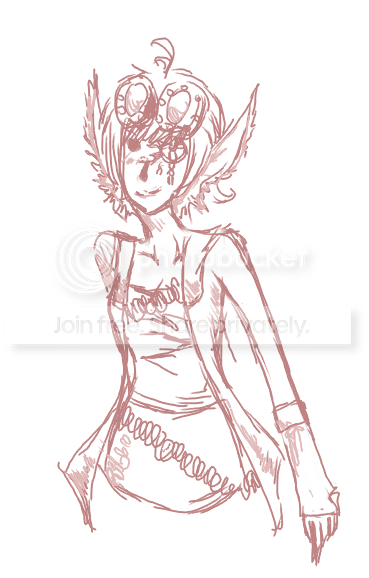^^^This is by General Ritsuka^^^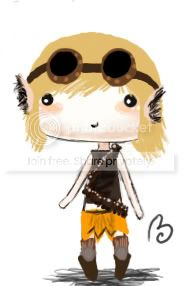^^^This is by Bipolar Masquerade^^^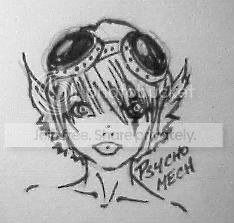^^^This is by Psychomech^^^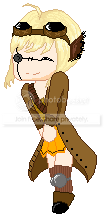^^^This is by Seductive Soverign^^^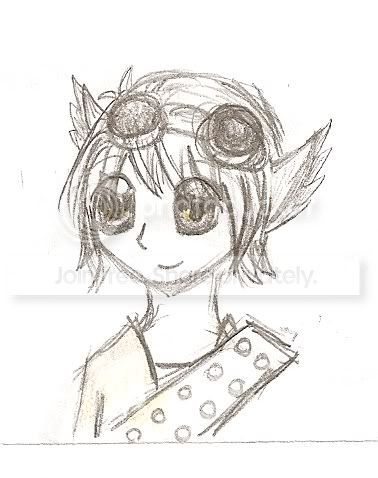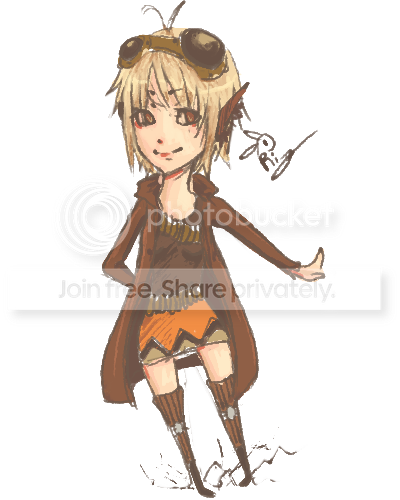^^^This is by R i b b u n^^^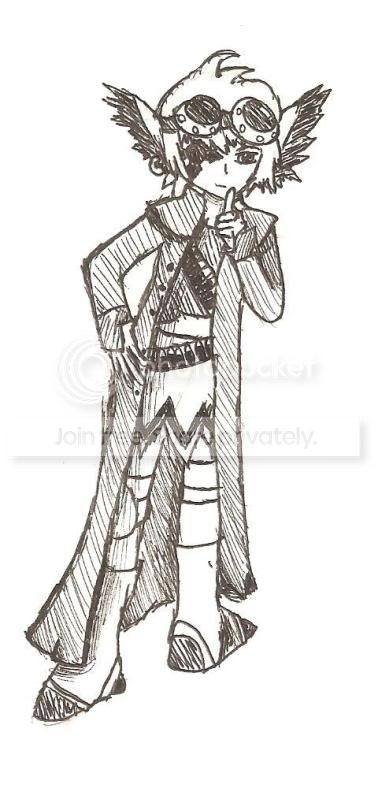^^^This is by Unbridled Originally^^^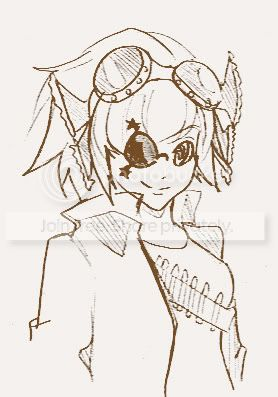^^^This is by Kira_Skullprince^^^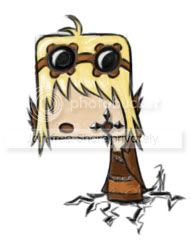^^^This is by Red_Head01^^^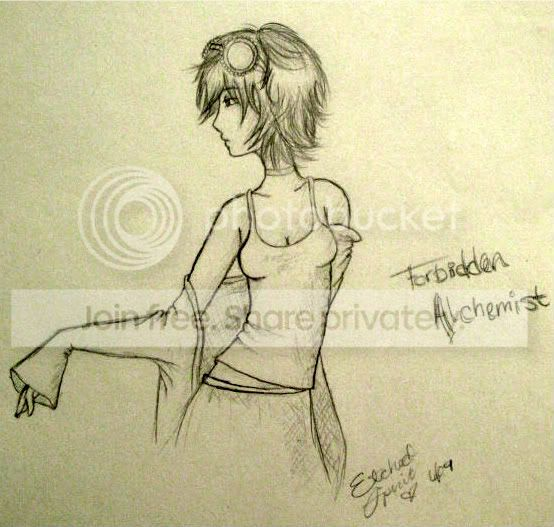^^^This is another picture made by EtchedSpirit^^^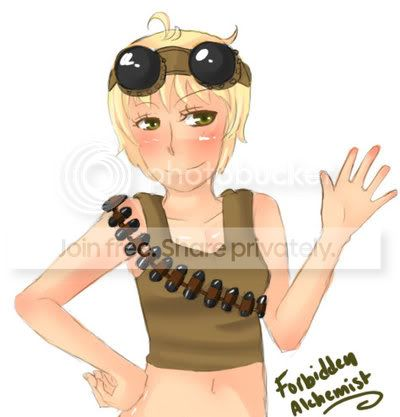^^^This is by Naturally Grown^^^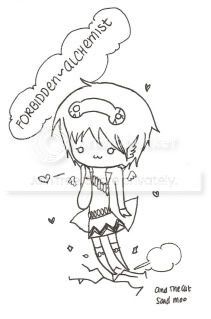^^^This is by and the cat said moo^^^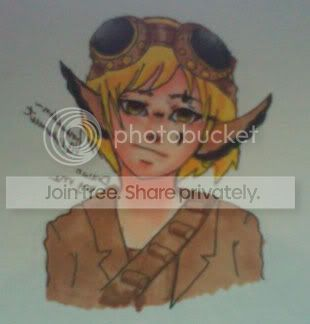^^^This art was made by me^^^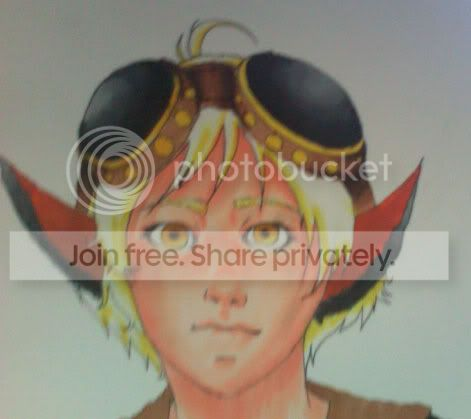^^^This is another art made by me^^^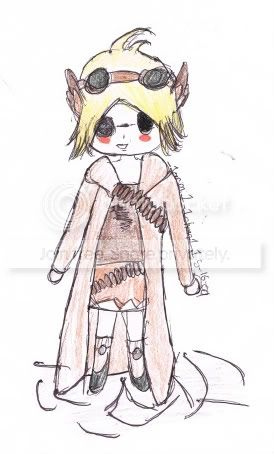^^^By _1arimi1_1chan1_1^^^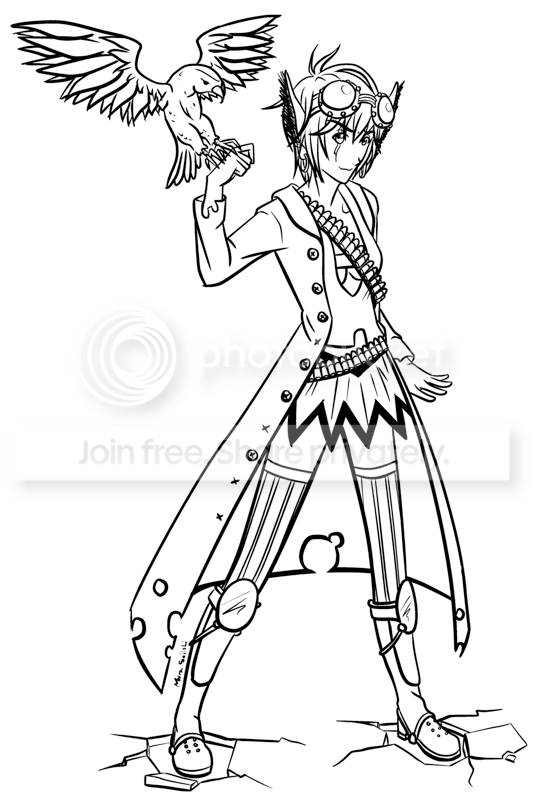^^^By Mura Soiishi^^^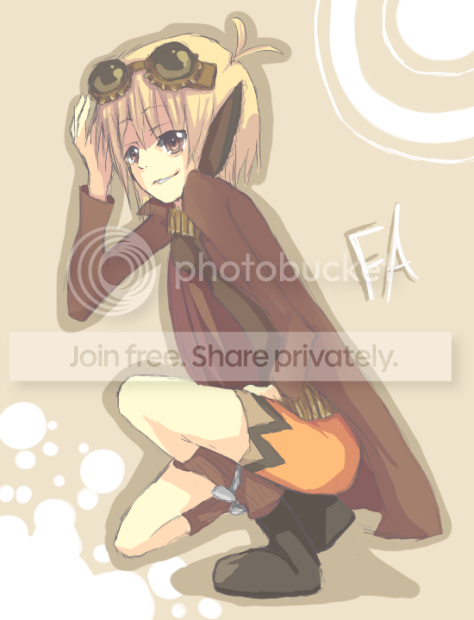^^^By Biancaemorappinrocker^^^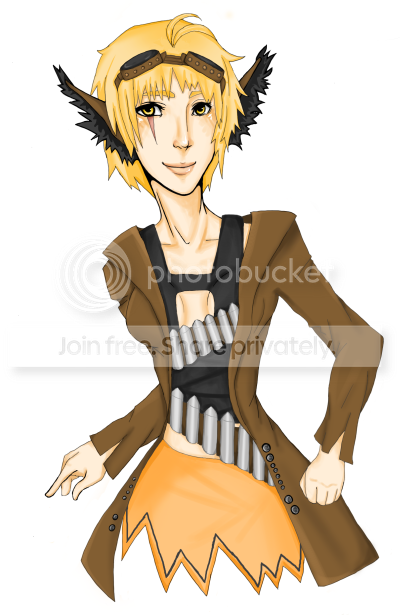^^^By L4g^^^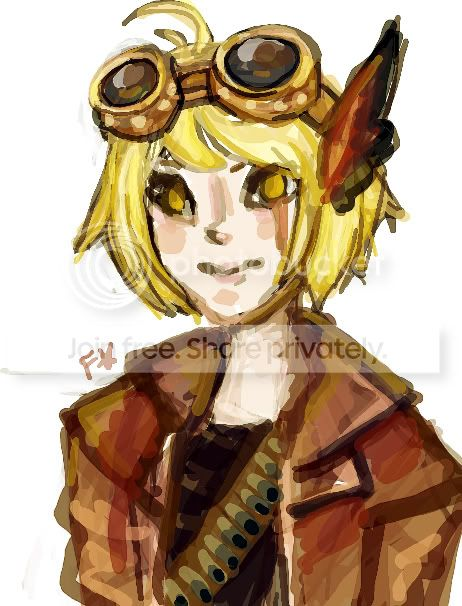^^^By Fawkes_Star^^^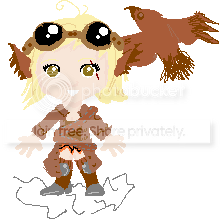^^^By x-slinkey-x^^^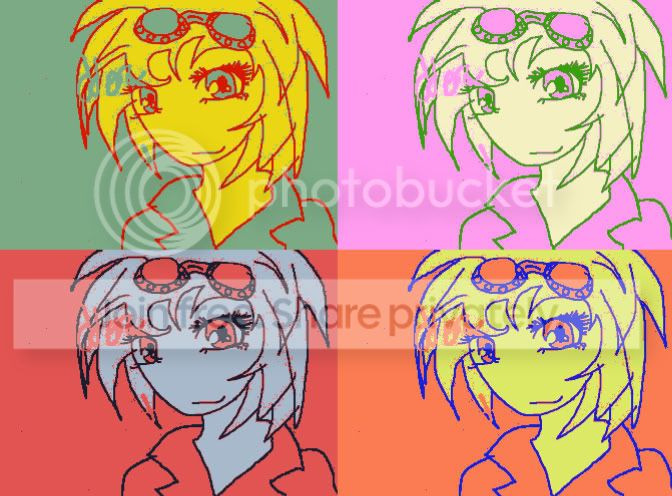^^^By AcidsRain^^^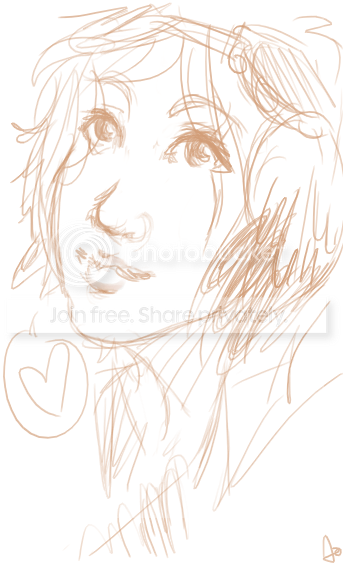^^^By Aokip^^^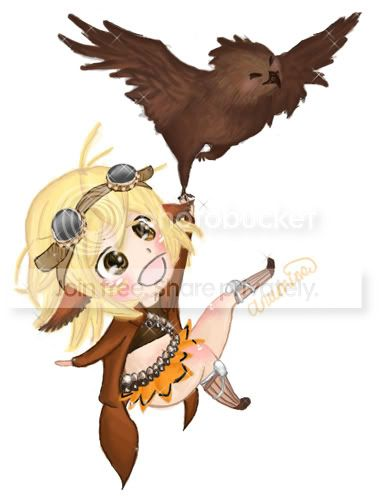^^^ By Anumine^^^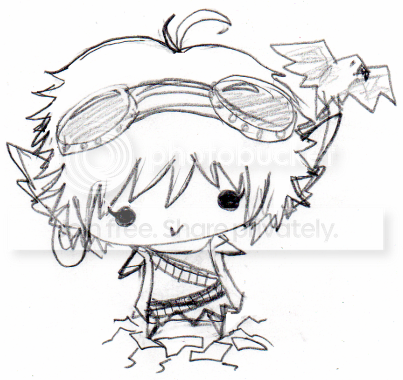^^^ By Kristina_kg^^^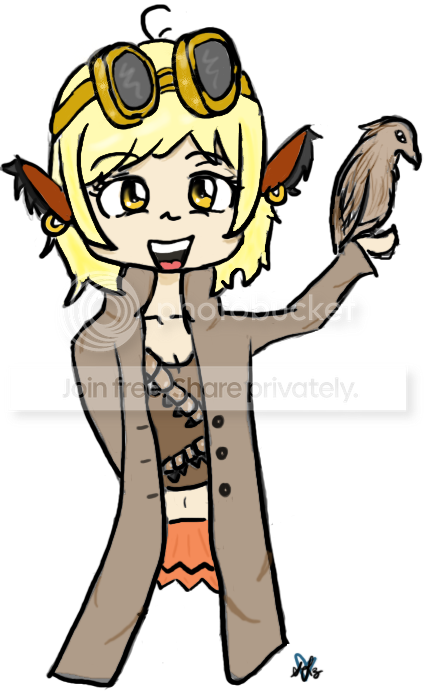^^^By spiffy_lil_z^^^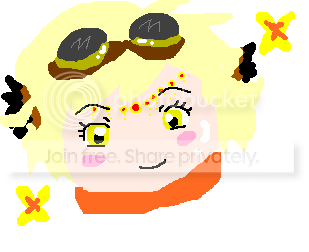^^^By Moo Princess^^^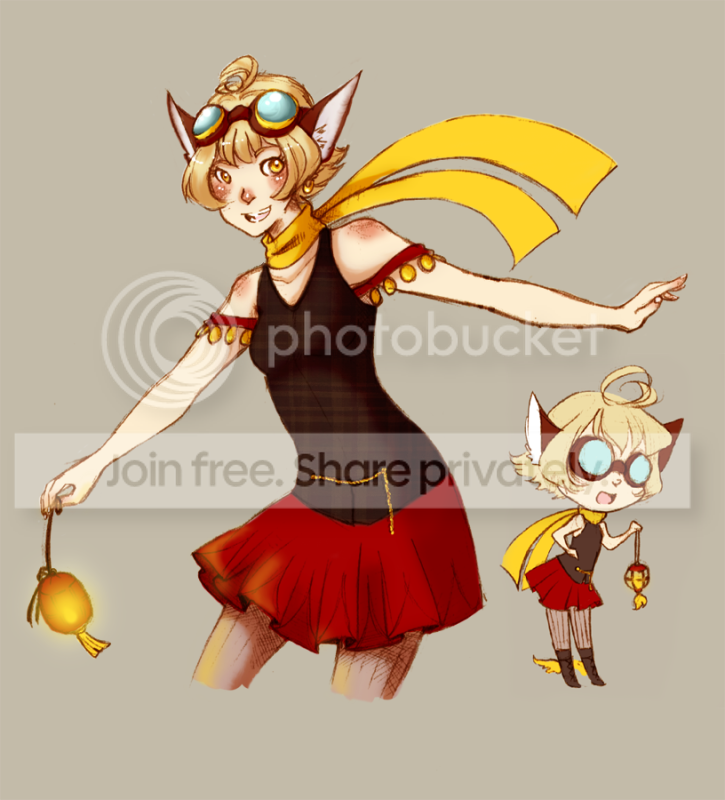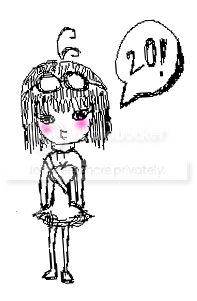^^^By Nope Sorry^^^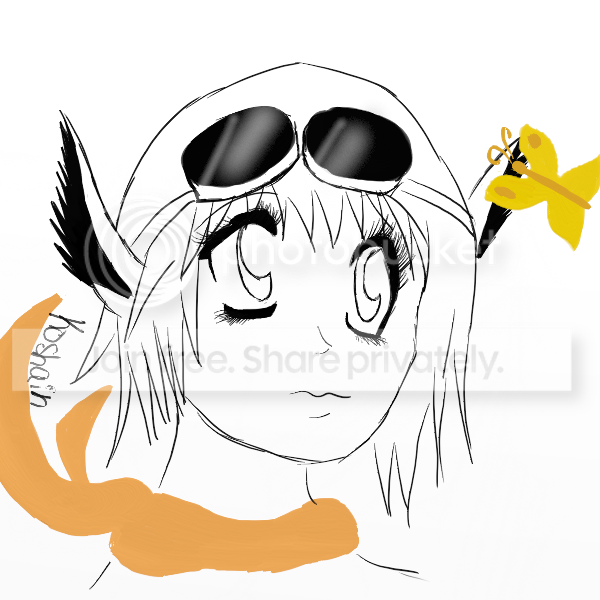^^^By Koshain^^^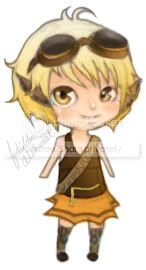^^^By Me^^^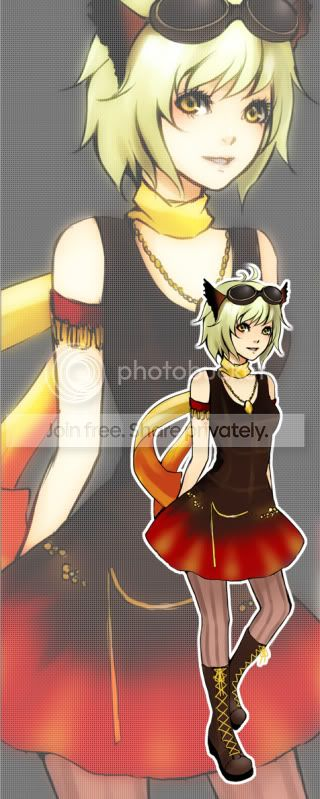^^^Commission by Lisemo on Deviantart^^^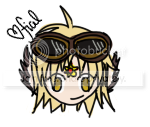^^^Fial^^^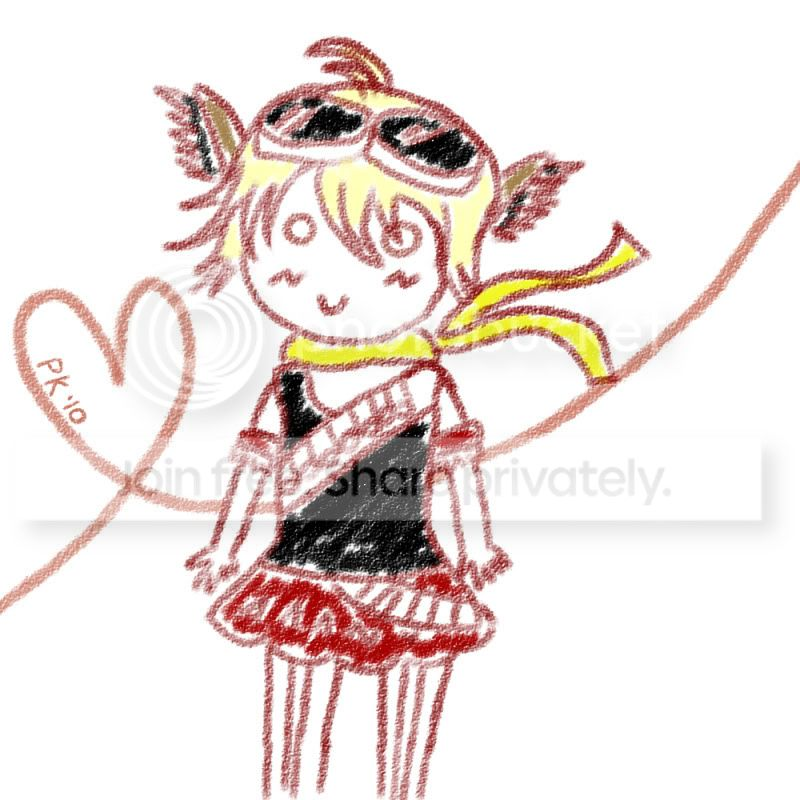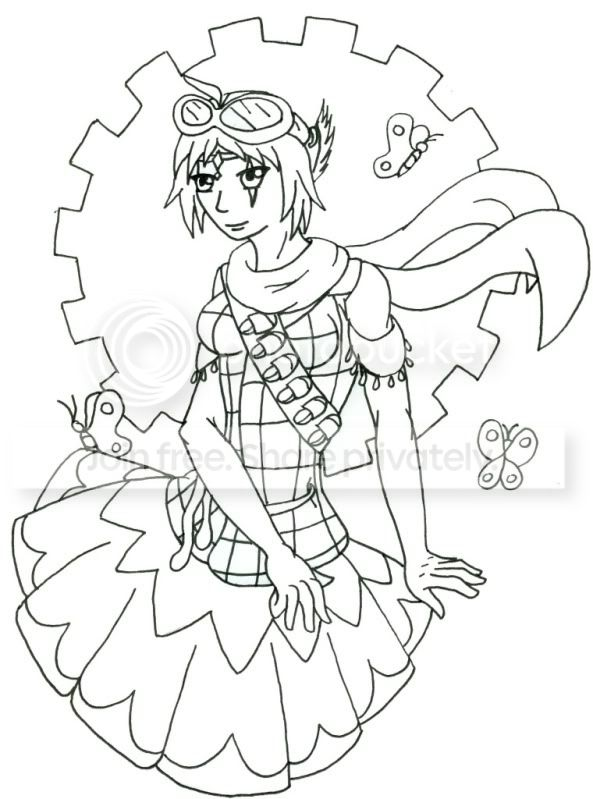^^^Dark Evili^^^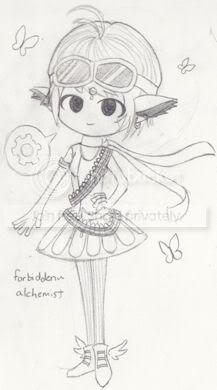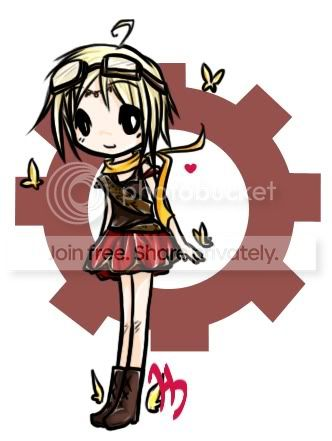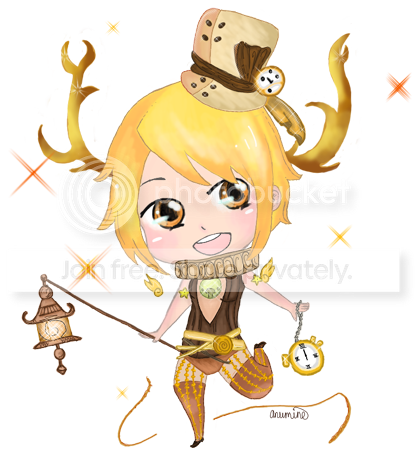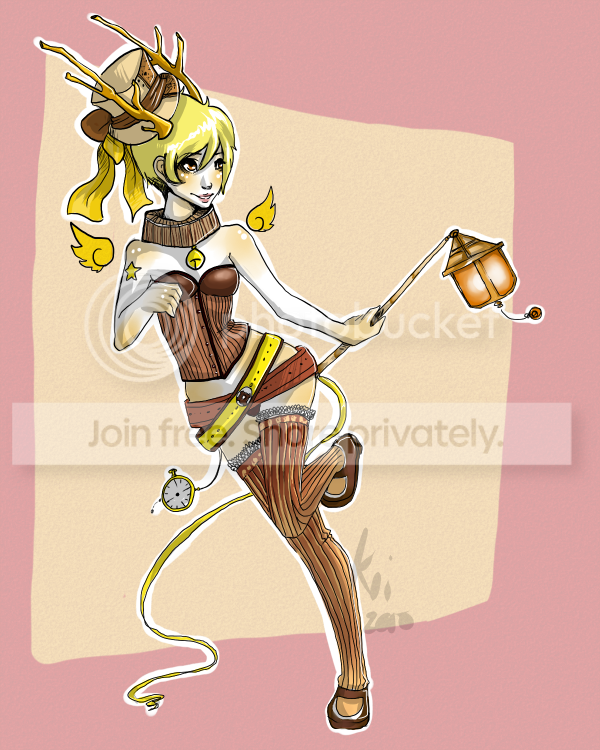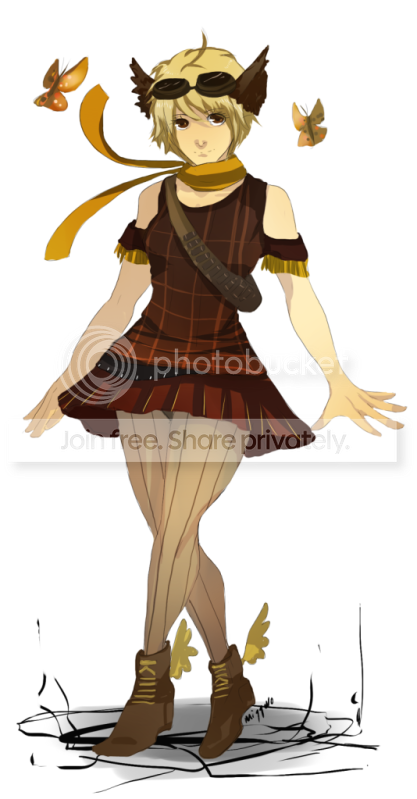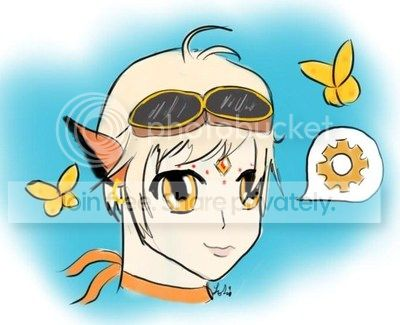^^^By Lokianna^^^^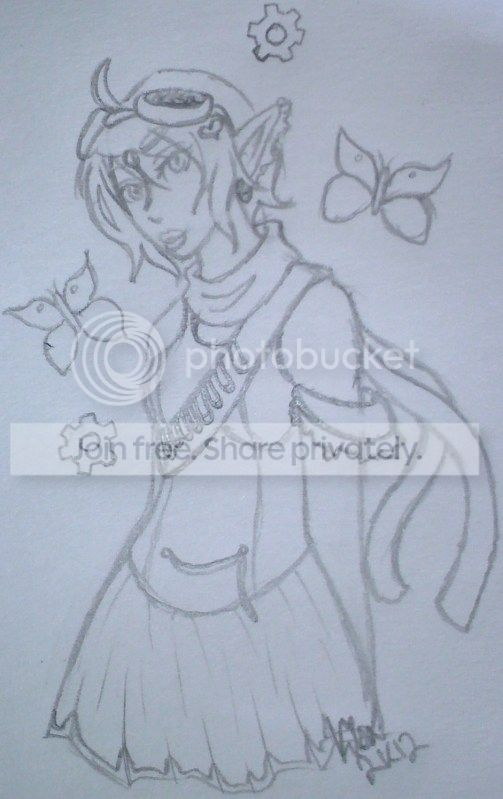^^^^By kisa_tiger13666^^^^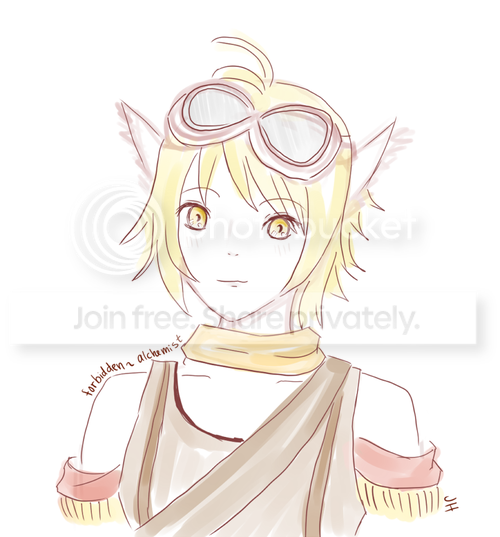^^^^By JellyfishHime^^^^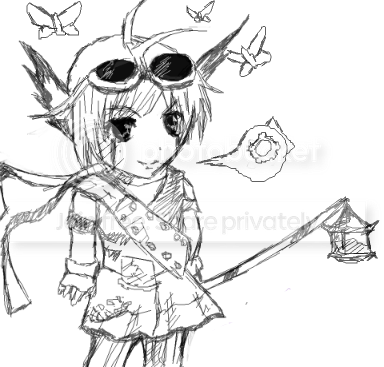^^^^By ken100shin from RMD^^^^Posted by: forbidden~alchemist Thu Jul 09, 2009 @ 07:56pmforbidden~alchemist
Community MemberPrev | Next»
Archive | Home

• [07/09/09 07:56pm]
• [05/30/07 09:37pm]
•